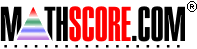Math Practice Online > free > lessons > Florida > 6th grade > Compare Mixed Values

## Compare Mixed Values

Compare fractions with decimals. Determine if one is less than, greater than, or equal to the other.

 Sample Problems for Compare Mixed Values Lesson for Compare Mixed Values

### This topic aligns to the following state standards

Grade 4: Num 1. Uses language and symbols (>, <, =) to compare numbers in the same form and in two different forms such as _ < 1.
Grade 4: Num 3. Compares and orders commonly used fractions and decimals to hundredths using concrete materials, drawings, and numerals.
Grade 5: Num 1. Uses symbols (>, <, =) to compare numbers in the same and different forms such as 0.5 < 3/4.
Grade 5: Num 3. Compares and orders commonly used fractions, percents, and decimals to thousandths using concrete materials, number lines, drawings, and numerals.
Grade 6: Num 1. Compares and orders fractions and decimals using graphic models, number lines, and symbols.
Grade 6: Num 2. Compares and orders fractions, decimals, and common percents.
Grade 7: Num 1. Compares and orders integers, fractions, decimals, numbers with exponents, and numbers expressed as percents or in scientific notation, including ordering on a number line.
Grade 8: Num 1. Compares and orders fractions, decimals, integers, and radicals using graphic models, number lines, and symbols.
Grade 6: Num 3. Knows whether numbers expressed in different forms are equal.
Grade 7: Num 3. Knows whether numbers expressed in different forms are equal.
Grade 9: Num 4. understands that numbers can be represented in a variety of equivalent forms, including integers, fractions, decimals, percents, scientific notation, exponents, radicals, absolute value, and logarithms

Copyright Accurate Learning Systems Corporation 2008.